# Powerbank vs Arduino¶

An external USB Battery, powerbank, is cheap. It has a lipo battery, a charging electronic, a step up converter to 5V. All in a convenient cheap package

The problem is, they are too intelligent. The step up converter is not hundred percent efficient. So the powerbank will shut it down if no load is applied.

You need to figure out the load, required to keep the powerbank running. By trial and error i found 90 mA was the minimum. An arduino uno is around 35. Adding 60 mA should be enough.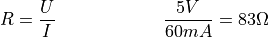and we will burn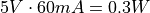Typical resisitors are 0.25 watt, so it is better to combine two of your arduino kit. One hundred and one two hundred Ohm resistor in parallel are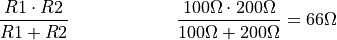which will draw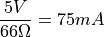plus the arduino uno that is enough. For smaller microcontrollers, two 100 Ohms will draw 100mA, but then the 0.25 Watt per Resistor is reached.

wasting power to heat is not very efficient. It is enough to pulse the load. Apply it every n seconds for a few milliseconds. Again the exact values are dependant on your powerbank.

If you know what to do, a simple blink circuit will do this. But we already have a microcontroller available and i don’t have the required condensators at hand.

We will require one transistor and one pin in of the arduino.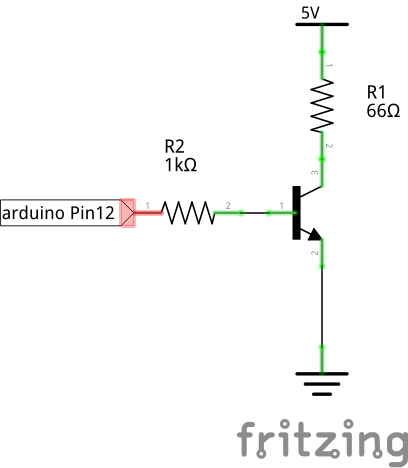Resistor R1 is the calculation from above. R2 protects the arduino

void setup() {
pinMode(12, OUTPUT);
}

void loop() {
DigitalWrite(12, HIGH);
delay(500);
digitalWrite(12, LOW);
delay(5000);
}


and we only use 10% of the permanent load of variant A